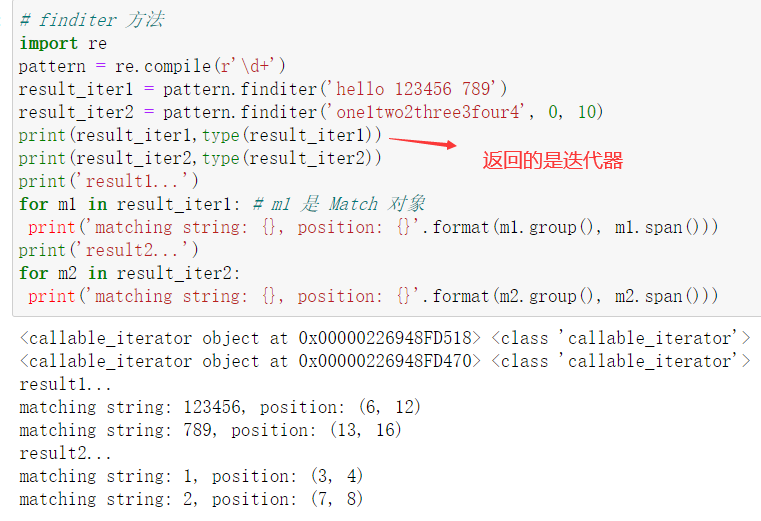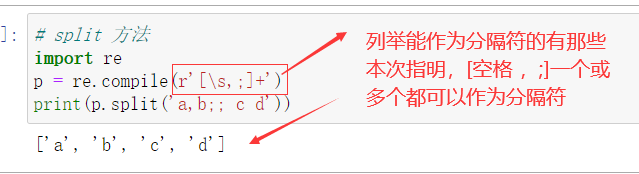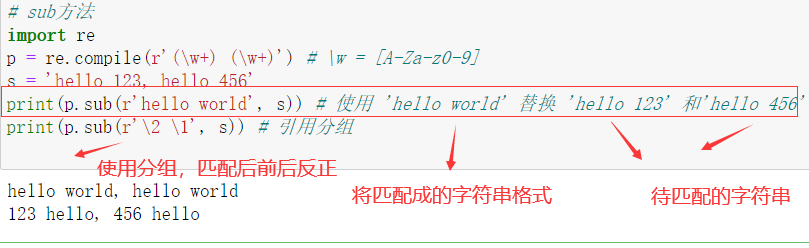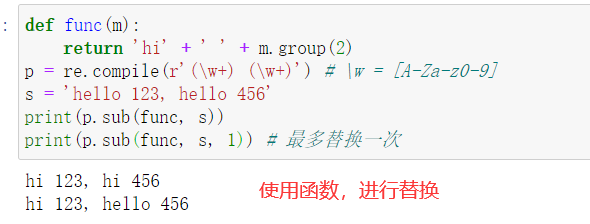# 正则表达式使用系列（五）finditer、split、sub 方法

## finditer 方法

``````	# -*- coding: utf-8 -*-
import re
pattern = re.compile(r'\d+')
result_iter1 = pattern.finditer('hello 123456 789')
result_iter2 = pattern.finditer('one1two2three3four4', 0, 10)
print(type(result_iter1))
print(type(result_iter2))
print('result1...')
for m1 in result_iter1: # m1 是 Match 对象
print('matching string: {}, position: {}'.format(m1.group(), m1.span()))
print('result2...')
for m2 in result_iter2:
print('matching string: {}, position: {}'.format(m2.group(), m2.span()))
``````## split 方法

split(string[, maxsplit])
maxsplit 用于指定最大分割次数，不指定将全部分割。

``````import re
p = re.compile(r'[\s,;]+')
print(p.split('a,b;; c d'))
``````## sub 方法

sub(repl, string[, count])
epl 可以是字符串也可以是一个函数：

count 用于指定最多替换次数，不指定时全部替换。

``````import re
p = re.compile(r'(\w+) (\w+)') # \w = [A-Za-z0-9]
s = 'hello 123, hello 456'
print(p.sub(r'hello world', s)) # 使用 'hello world' 替换 'hello 123' 和
'hello 456'
print(p.sub(r'\2 \1', s)) # 引用分组
def func(m):
return 'hi' + ' ' + m.group(2)
print p.sub(func, s)
print p.sub(func, s, 1) # 最多替换一次
``````

epl 参数是字符串epl 参数是函数